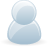# Support Forum

or## Calibration

2593 Views - Created 03/10/2016

03/10/2016

Posted by:
ajay100Location:
Warragul, Victoria Australia

03/10/2016

Posted by:
andrewLocation:
United Kingdom

If you are using our ADCPi python library to read the values from your ADC Pi then the easiest way to make it work for 60V input would be to modify the read_voltage() function within the library to use a multiplier based on the voltage divider you are using.

The read_voltage() function contains the following code on line 116:

(raw * (self.__lsb / self.__pga)) * 2.471)

The 2.471 is the multiplier for the voltage divider. This is calculated based on the maximum input voltage divided by the maximum output voltage. On the ADC Pi, the voltage divider contains a 10K and 6K8 resistor and the maximum voltage the ADC chip can read is 2.048V. Using our voltage divider calculator with R1 at 10000, R2 at 6800 and Output as 2.048 gives an input voltage of 5.05976V.

5.05976 / 2.048 = 2.47058 which in the ADC Pi library is rounded to 2.471.

The code Andrew was using contained an early version of our library that had an incorrect value for the multiplier of 2.448579823702253 which we have since fixed.

If you are using external 200K resistors then that combined with the existing 10K will give a 210K on the input side of the divider. This will give a maximum voltage of 65.29506V which can be divided against the 2.048 output voltage to give us a new multiplier.

65.29506 / 2.048 = 31.8824

Updating the ADC Pi library so the code above now contains the new multiplier should give you the correct voltage readings when using the read_voltage() function. This should be simpler than the method Andrew used.

(raw * (self.__lsb / self.__pga)) * 31.8824)

The accuracy of the readings will be based on the tolerances of the resistors so if you need to make the circuit more accurate you will need a calibrated voltage source to measure against and then comparing the voltage source to the value being recorded you can work out an error correction value for each input channel.

24/10/2016

Posted by:
ajay100Location:
Warragul, Victoria Australia

Thanks so much for your excellent, detailed and speedy response. It all makes sense, particularly as I was stuck on the values used in the early library. I've now got the code working nicely.